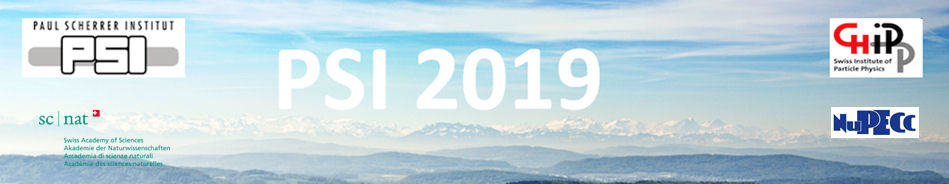#Physics of fundamental Symmetries and Interactions - PSI2019

Oct 20 – 25, 2019
PSI
Europe/Zurich timezone

## The CKM unitarity problem: A trace of new physics at the TeV scale?

Oct 24, 2019, 4:30 PM
30m
WHGA/001 - Auditorium (PSI)

Invited Talk

### Speaker

Prof. Zurab Berezhiani (Univ. L'Aquila and LNGS)

### Description

After the recent high precision determinations of $V_{us}$ and $V_{ud}$, the first row of the CKM matrix shows more than $4\sigma$ deviation from unitarity. Two possible scenarios beyond the Standard Model can be investigated in order to fill the gap. If a 4th quark $b'$ participates in the mixing, with $\vert V_{ub'} \vert \simeq 0.04$, then its mass should be no more than 6 TeV or so. A different solution can come from the introduction of the gauge horizontal family symmetry acting between the lepton families and spontaneously broken at the scale of about 6 TeV. Since the gauge bosons of this symmetry contribute to muon decay in interference with Standard Model, the Fermi constant is slightly smaller than the muon decay constant so that unitarity is recovered. Also the neutron lifetime problem, that is about $4\sigma$ discrepancy between the neutron lifetimes measured in beam and trap experiments, is discussed in the light of the the novel determinations of the CKM matrix elements.

### Primary author

Prof. Zurab Berezhiani (Univ. L'Aquila and LNGS)